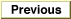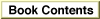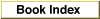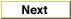Inside Macintosh: Devices /
Chapter 7 - Serial Driver / Serial Driver Reference
Serial Driver Routines /

### SerReset

You can use the `SerReset` function to reset the serial port drivers and configure the port for a specified transmission rate and character frame.

```FUNCTION SerReset (refNum: Integer; serConfig: Integer): OSErr;
```
`refNum`
The driver reference number of the serial output driver.
`serConfig`
A 16-bit value that specifies the configuration information.
##### DESCRIPTION
The `SerReset` function resets the output and input device drivers for the serial port, and also configures the port according to the format of the `serConfig` parameter shown in Figure 7-3.

Figure 7-3 The `serConfig` parameter format[Missing image]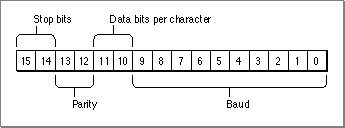You can use the following constants to set the values of the bit fields in the `serConfig` parameter:

```CONST
baud300     = 380;      {300 baud}
baud600     = 189;      {600 baud}
baud1200    = 94;       {1200 baud}
baud1800    = 62;       {1800 baud}
baud2400    = 46;       {2400 baud}
baud3600    = 30;       {3600 baud}
baud4800    = 22;       {4800 baud}
baud7200    = 14;       {9600 baud}
baud9600    = 10;       {3600 baud}
baud14400   = 6;        {14400 baud}
baud19200   = 4;        {19200 baud}
baud28800   = 2;        {28800 baud}
baud38400   = 1;        {38400 baud}
baud57600   = 0;        {57600 baud}
stop10      = 16384;    {1 stop bit}
stop15      = -32768;   {1.5 stop bits}
stop20      = -16384;   {2 stop bits}
noParity    = 0;        {no parity}
oddParity   = 4096;     {odd parity}
evenParity  = 12288;    {even parity}
data5       = 0;        {5 data bits}
data6       = 2048;     {6 data bits}
data7       = 1024;     {7 data bits}
data8       = 3072;     {8 data bits}
```
For example, the default setting of 9600 baud, eight data bits, two stop bits, and no parity bit is equivalent to passing the following value in the `serConfig` parameter:

```	baud9600 + data8 + stop20 + noParity.
```
This value has a binary representation of `1100110000001010` and a hexadecimal representation of `\$CC0A`.

##### ASSEMBLY-LANGUAGE INFORMATION
The `SerReset` function is equivalent to a Device Manager control request with a `csCode` value of 8. You pass the `serConfig` parameter in the `csParam` field (`csParam` = `serConfig`).

##### RESULT CODES noErr0No error